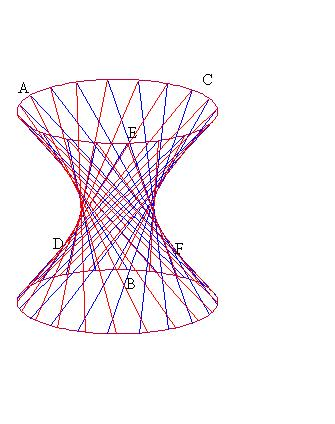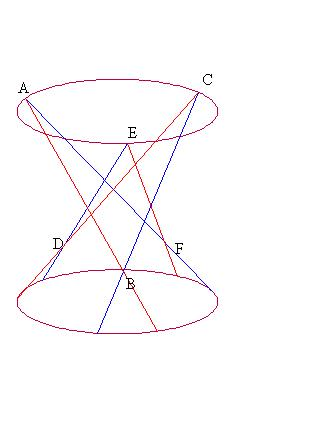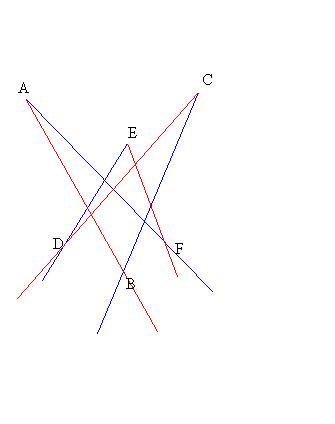# (after proof in Hilbert and Cohn-Vossen- Geometry and the Imagination.)

Preliminaries: First note some properties for the surface of a hyperboloid of one sheet.

Such a surface is formed by rotating an hyperbola around its separating axis of symmetry.
This surface has two distinct partitions by a non-intersecting lines with the property any member of one partition meets each member of the other family in a unique point.

This can be understood by considering the surface as arising by taking a circular cylinder formed by two circles and the lines on the surface parallel to the axis of the cylinder and  rotating one of the circles while keeping the  points of intersection of the lines with the circles fixed. This will  lead to a hyperboloid of one sheet as the new surface and the lines on that surface will still be non-intersecting- thus creating the partition by these lines. Rotating the circle the same amount but in the opposite direction gives the other partition and should make it easy to see why these families will intersect so completely.Now consider a hexagon that is determined by six lines alternately chosen from these two line partitions on the surface of a hyperboloid of one sheet.

Suppose A,B,C,D,E, and F denote the vertices of the hexagon with sides, AB, BC,CD,DE,EF, FA.
Consider the three diagonal line formed by joining opposite vertices: AD, BE and CF. [See figures below.]

Claim: The three diagonal lines, AD, BE, and CF, all meet at a single point.Proof of claim:
Notice that AB and DE must meet since they are from "opposite" families that partition the hyperboloid. So ABDand E all lie in the plane determined by these two line.
Thus AD and  BE lie in the same plane and must have a point of intersection, X, in that plane.

Similarly AD and CF lie in the same plane determined by ADC and F and must have a point of intersection, Y, in that plane.
Likewise, BE and CF all lie in a single plane determined by BEC and F and also must have a point of intersection, Z, in that plane.

If the points X, Y and Z are distinct then they determine a plane. But X and Y both lie on the line AD, while Y and Z both lie in the line CF. But this means that AD, and CF lie in the plane determined by X, Y and Z , and likewise ,BE lies in this same plane.
But the 6 points A,B, C, D, E, and F do not lie in a plane! This is a contradiction. So it must be that the points X, Y and Z are not distinct, but in fact are the same, X = Y = Z, or the three diagonal lines for a hexagon on the surface of a hyperbaloid of one sheet all meet at a single point.

Outline of proof of Brianchon's Theorem:
The projection of a hyperboloid of one sheet onto a plane from a single point in space will give a curve in the plane determined by all the points that are covered by exactly one points. That curve will be a conic, and tangent lines to that conic will correspond to lines from the two line partitions of the hyperboloid.  [Include demonstrating figure here.]

In fact, any conic in a plane can be obtained by such a projection. Thus the lines of a hexagon that are tangent to a conic in the plane can be assumed to be the projections of a hexagon formed by six lines chosen alternately from the line partitions of a hyperboloid of one sheet. Since the diagonals of the hyperboloid's hexagon meet at a single point, and projection preserves lines and points, the diagonals of the original hexagon ( which is the projection of the hyperboloid's hexagon)  also meet in a single point.

EOP Outline.